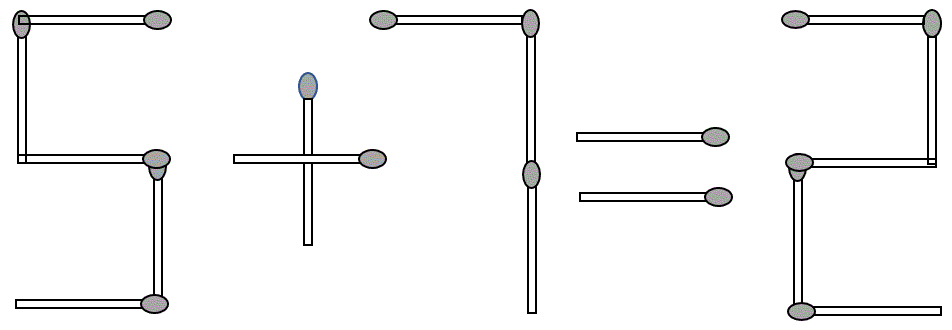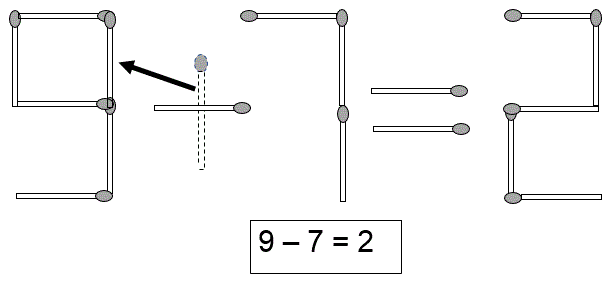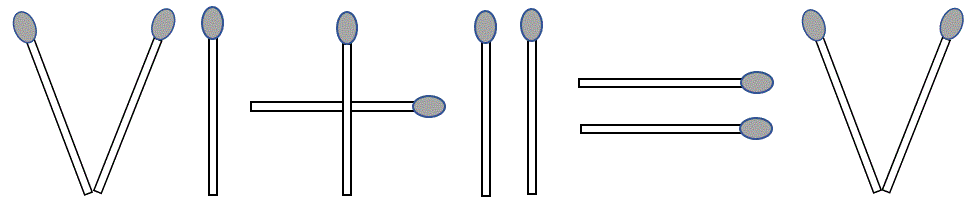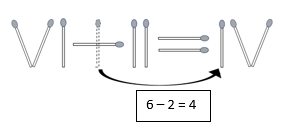Select Page

1. This matchstick image of an equation (6 – 4 = 3) is incorrect. Can you correct the equation by moving just one matchstick?  All matchsticks must be used.### Solution2. This matchstick image of an equation (5 + 7 = 2) is incorrect. Can you correct the equation by moving just one matchstick? All matchsticks must be used.### Solution3. This matchstick image of a Roman numeral equation (6 + 2 = 5) is incorrect. Can you correct the equation by moving one matchstick?### Solution## Feedback

There are more than one way of doing these puzzles and may well be more than one answer.  Please let me and others know what alternatives you find by commenting below.  We also welcome general comments on the subject and any feedback you’d like to give.   If you have a question that need a response from me or you would like to contact me privately, please use the contact form.

## Get more puzzles!

If you’ve enjoyed doing the puzzles, consider ordering the book; 150+ of the best puzzles in a handy pocket sized format. Click here for full details.

### Share This

Share this post with your friends!